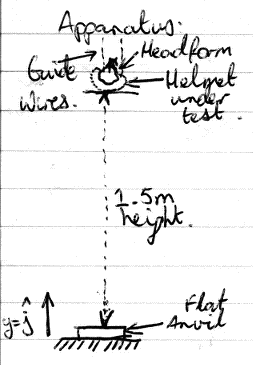This afternoon, I managed to stumble on one of my old text books from my first years studying Electrical Engineering at university. In the first year, we future engineers are all one big happy family, with civil, electronics and other groups, all lumped together. So we study the same things, including physics.

For me, studying at QUT in 2004, the text book we were told to read was Physics for Scientists and Engineers with Modern Physics , Serway and Jewett, 6th Edition (International student edition, ISBN 0-534-40949-0 ). In reading this, I realised I had made an error in my earlier analysis of this test case.

So we make the same assumptions as before. That doesn’t change. We fix our earlier incorrect equation, and re-calculate based on that. If someone does happen to notice something amiss, feel free to let me know. If you’re not a Hackaday.io user, I can be contacted a number of ways.

Another difference is that the CPSC tests use a headform mounted to a carriage that runs along a single rail, whereas Australian Standards testing appear to use the dual-guide-wire technique judging from the crash.org.au videos, one of which is here (Sorry guys, I’d link to the page, but then 1999 called asking for their proprietary Flash Player plug-in back).

Thus, without further ado, I present my re-analysis.

First, a diagram of the test set-up.So y increases with distance from the anvil mounted on the floor. The guide wires are assumed to present negligible resistance, and we’ll ignore wind resistance.

Annoyingly, I can’t copy and paste the table from earlier, so please refer to my previous post on this for the assumed values.

Taking the correct equation for the height over time, and trying to solve for when the helmet meets the anvil, the impact time, we get the following equation:$0={1 \over 2}(-9.8)(t_I)^2 + 0t_I + 1.5$ which re-arranged, gives:$t_I = \sqrt {-1.5 \over {({1 \over 2}) \times -9.8}} = \sqrt {-1.5 \over -4.9} = 0.553283$

So a flight time of 553.283 milliseconds. In that time, it accelerates by -9.8m/s every second, so we can work out the impact velocity.$v_I=at_I=-9.8 \times 0.553283 = -5.422$ We can work out the momentum at that the time of impact.$p_I = mv_I = -27.111$ Our worst case is an elastic collision, so we can describe the momentum after impact as being the momentum at impact, in the opposite direction:$p_A=-p_I=27.110$ This gives us a change in momentum as follows:$\Delta p = p_A - p_I = 27.110 - (-27.110) = 54.222$ We can’t model an instantaneous change, so let’s assume it happened all in a millisecond. That gives us the following force after impact:$F_A={\Delta p \over \Delta t} = {54.222 \over {10^{-3}}} = 54221.767$ Given the headform/helmet mass, that gives us an upward acceleration of:$a_A={F_A \over m}=10844.353 = 1106.567 g$ If the other scenario didn’t kill our cyclist, this definitely would.